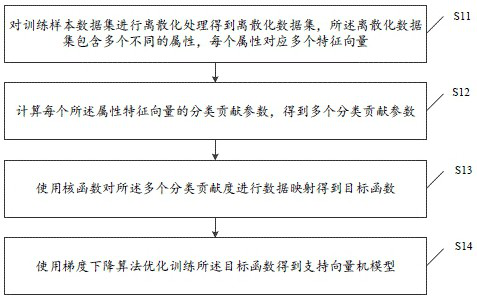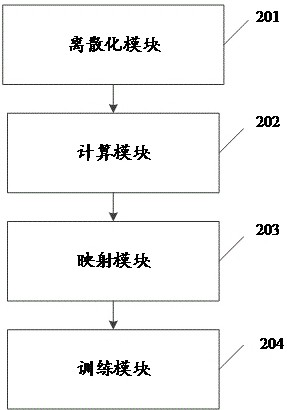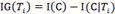# Multi-dimensional training method and device of support vector machine

## A technology of support vector machine and training method, which is applied in the field of multi-dimensional training method and device of support vector machine, can solve problems such as difficult to mine data relationship or connection, low analysis accuracy, complex operation, etc., and achieve improved classification and analysis Ability to improve the effect of linear separability

Pending Publication Date: 2022-03-15
GUANGDONG POWER GRID CO LTD +1
0 Cites 0 Cited by

## AI-Extracted Technical Summary

### Problems solved by technology

 However, the current commonly used training methods have the following technical problems: because the training data requires the user to manually adjust the relationship between each data, the operation is complicated, and the SVM can only be ...
View more

### Method used

In the present embodiment, the embodiment of the present invention provides a kind of multi-dimensional training method of support vector machine, and its beneficial effect is: the present invention can first carry out discretization processing to training sample data set and obtain discretization data set; Then calculate the classification contribution parameter of each attribute eigenvector; then use kernel function to carry out data mapping to a ...
View more

## Abstract

The invention discloses a multi-dimensional training method and device for a support vector machine, electronic equipment and a computer readable storage medium, and the method comprises the steps: carrying out the discretization of a training sample data set, and obtaining a discretized data set, the discretized data set comprises a plurality of different attributes, and each attribute corresponds to a plurality of feature vectors; calculating a classification contribution parameter of each attribute feature vector to obtain a plurality of classification contribution parameters; performing data mapping on the plurality of classification contribution degrees by using a kernel function to obtain a target function; and optimizing and training the objective function by using a gradient descent algorithm to obtain a support vector machine model. According to the method, different dimension data are mapped through the kernel function, the data gain weight can be determined, the linear separable effect of the mapped dimension data can be improved, and then the classification and analysis capability of the SVM can be improved.

Application Domain

Kernel methodsCharacter and pattern recognition

Technology Topic

Data linesDiscretization +11

## Image

•••## Examples

• Experimental program(1)

### Example Embodiment

Next, the technical solutions in the embodiments of the present invention will be described in connection with the drawings of the embodiments of the present invention, and it is understood that the described embodiments are merely the embodiments of the present invention, not all of the embodiments. Based on the embodiments in the present invention, all other embodiments obtained without creative labor are not made in the premise of creative labor.
 The currently common training methods have the following technical problems: Since the data of the training requires the user to manually adjust the relationship between the data, the operation is complex, and the SVM can only train the data relationship input by the user, it is difficult to excavate data between different dimensions. Relationship or contact, the accuracy of analysis after training is low, does not meet the actual needs.
 In order to solve the above problems, a multi-dimensional training method of the present application embodiment will be described in detail with reference to the following specific embodiments.
 Refer figure 1 The flow schematic diagram of a multi-dimensional training method for supporting a vector machine is shown in an embodiment of the present invention.
 Among them, as an example, the multi-dimensional training method of the support vector can include:
 S11, discretizing the training sample data set to obtain a discrete data set, the discrete data set contains a plurality of different properties, each attribute, corresponding to multiple feature vectors.
 In an embodiment, by discreating the data, each of the data can be separated from each other, thereby convenient determining connection between the respective data between different dimensions or different locations.
 Among them, as an example, the discrete processing may include the following sub steps:
 Sub-step S111, sort the plurality of feature vectors corresponding to each attribute according to the size of the vector value, to obtain the arrangement of the arrangement corresponding to each attribute.
 Sub-step S112, based on the preset division number divides the arrangement feature vector into a plurality of vector regions, wherein each vector interval corresponds to an attribute feature vector.
 Alternatively, it is assumed that Q is a collection of samples. Q = { Y) | 1
 S is a discrete data set by the sample set Q through discrete treatment. S = { Y) | 1
 Feature vectors corresponding to each attribute in the sample (1
 X is a feature attribute collection, x = { | 1 } It is a high dimensional feature vector.
 N is the number of samples.
 Y is the classified property.
 T is a collection of attributes , M: attribute characteristics.
 T is attribute appear, :Attributes does not appear
 Subset after the collection of characteristic attributes quantity. Attributes There are V different values, divide the discrete data set S is divided into V subset,.
 Subset Belong to the class quantity. Sample collection Q is divided into based on the classification properties y. For the subset quantity.
 S12, calculate the classification contribution parameters of each of the attribute feature vectors to obtain a plurality of classification contribution parameters.
 In an alternative embodiment, the classification contribution parameters, including: the desired information of the sample classification, variable value, condition entropy, information gain weight, classification contribution rate, and physical distance;
 The calculation of the desired information of the sample classification is as shown in the following formula:
 , The number of subsets included in the attribute, For the subset quantity;
 The calculation of the variable value probability is as shown in the following formula:
 ;
 The calculation of the conditional entropy is shown in the following formula:







 ;
 Division for feature attributes, each subsequence quantity;
 The calculation of the information gain weight is shown in the following:


 ;
 The calculation of the classification contribution rate is shown in the following formula:

 Collection of attributes;
 The calculation of the inter-entity distance is shown in the following formula:
 .
 S13, using a core function to perform data mapping of the plurality of classification contributions to obtain the target function.
 In one of the embodiments, step S13 can include the following sub steps:
 Sub-step S131, use a core function to linearly mapping each of the classification contributions to obtain a mapping function of each of the classification contributions.
 Sub-step S132, calculate the inner product of each of the classification contributions by the mapping function to obtain a target function corresponding to each of the classification contributions.
 In an embodiment, the core function comprises: a Gaussian nuclear function;
 The Gaussian nuclear function is shown in the following:
 .
 In yet another embodiment, the kernel function further includes: TS core function;
 The TS core function is shown in the following formula:
 .
 In one embodiment, multiple kerging can be used to linearly mapping each classification contribution, and the specific mapping function may not be required during mapping, and the mapping function is not required.
 In an embodiment, the support vector machine (SVM) can be as follows:

 Specifically, according to the definition of the nuclear function: assuming that H is the mapping feature space, T is the original feature space. If there is a mapping from T to H : T -> h, make all X, w T, function To meet the conditions , Then For the nuclear function, For the mapping function, for Introduction.
 As can be seen from the SVM classifier FF (X), the subsequent processing needs to be obtained from the meta of W and X. Then W and X are mapped after the Gaussian mapping function And the result of obtaining and The results of the inner product. Therefore, use the core function to process the variable X and W mapping, and avoid calculating and Afterwards and Multiplied.
 S14, optimize training of the target function using a gradient drop algorithm to obtain the support vector machine model.
 In an embodiment, the support vector machine (SVM) is used in the case where data linear can be used. Most of the current data is high dimension data, high dimensional data is generally nonlinear score in space, using a nuclear function to linearly mapping X, and the target function after the nuclear is a nonlinear promotion of the SVM classifier.
 In an embodiment, step S14 may include the following sub steps:
 The sub-step S141 converts the target function into optimization problems.
 Sub-step S142, the gradient of the optimization problem.
 Sub-step S143, iterative update gradient gets a support vector machine model.
 Among them, the conversion of optimization problems is shown in the following formula:

 Is a hinge loss function;

 Vector w and vector Introduction;
 The calculation of the gradient is shown in the following formula:


 R is an iterative number, Is 0 vector, It is the sample point that is randomly selected randomly, Is the weight parameters of the R-1 iteration;
 The calculation of the iterative update is shown in the following:


 The calculated support vector machine model is shown in the following:
 .
 In the present embodiment, its optimization training is to obtain F (x) after the nuclear functions in the support vector machine (SVM), and then optimize F (X), and to use MINL (W) Gradient solving method, solving W.
 In an embodiment, the above iterative update W refers to how W How to update the value during iteration. After the iterative cycle is completed, the W vector value can be solved. Assume that the solution at this time is Will solve Bring f It is obtained from the nuclear SVM classifier calculation model.
 In the present embodiment, the embodiment of the present invention provides a multi-dimensional training method for supporting the vector, wherein the present invention can first discretize the training sample data set to obtain discrete data sets; then calculate each Classification contribution parameters of the attribute feature vector; then use the core function to perform data mapping for multiple classification contributions; finally use the gradient drop algorithm to optimize the target function to obtain the support vector machine model, since the present invention passes the nuclear function to different dimensions The data is mapped, and the data gain weights can be determined and the effect of the dimension data linear can be improved, and the SVM classification and analysis capabilities can be improved.
 The embodiment of the present invention also provides a multi-dimensional training device for supporting vector machines, see figure 2 A structural diagram of a multi-dimensional training apparatus for supporting a vector machine according to an embodiment of the present invention is shown.
 Among them, as an example, the multi-dimensional training device of the support vector can include:
 The discrete module 201 is used to discretize the training sample data set to obtain a discrete data set, the discrete data set contains a plurality of different attributes, each attribute corresponding to multiple feature vectors;
 The calculation module 202 is used to calculate the classification contribution parameters of each of the attribute feature vectors to obtain multiple classification contribution parameters;
 The mapping module 203 is configured to use a core function to data mapping of the plurality of classification contributions to obtain a target function;
 Training module 204 for optimizing training of the target function using a gradient drop algorithm to obtain a support vector machine model.
 Alternatively, the discrete treatment is specifically:
 Sort each attribute according to the size of the vector value, to obtain the arrangement of each property corresponding to each attribute;
 The arrangement feature vector is divided into a plurality of vector regions based on the preset division number, wherein each vector interval corresponds to an attribute feature vector.
 Alternatively, the classification contribution parameters include: the desired information of the sample classification, the variable ratio, the conditional entropy, the information gain weight, the classification contribution rate, and the physical distance;
 The calculation of the desired information of the sample classification is as shown in the following formula:
 , The number of subsets included in the attribute, Is the i-th attribute feature vector in the property;
 The calculation of the variable value probability is as shown in the following formula:
 ;
 The calculation of the conditional entropy is shown in the following formula:







 ;
 Division for feature attributes, each subsequence quantity;
 The calculation of the information gain weight is shown in the following:


 ;
 The calculation of the classification contribution rate is shown in the following formula:

 Collection of attributes;
 The calculation of the inter-entity distance is shown in the following formula:
 .
 Alternatively, the mapping module is also used in:
 Use the core function to linearly mapping each of the classification contributions to obtain a mapping function of each of the classification contributions;
 With the mapping function, each of the category contributions is calculated, and the target function corresponding to each of the classification contributions is obtained.
 Alternatively, the core function includes: Gaussian nuclear function;
 The Gaussian nuclear function is shown in the following:
 .
 Alternatively, the kernel function further includes: TS core function;
 The TS core function is shown in the following formula:
 .
 Alternatively, the training module is also used in:
 Convert the target function to optimization problems;
 Seeking the gradient of the optimization problem;
 Iterative Update Gradient Get Support Vector Machine Model;
 Among them, the conversion of optimization problems is shown in the following formula:

 Is a hinge loss function;

 Vector w and vector Introduction;
 The calculation of the gradient is shown in the following formula:


 R is an iterative number, Is 0 vector, It is the sample point that is randomly selected randomly, Is the weight parameters of the R-1 iteration;
 The calculation of the iterative update is shown in the following:


 The calculated support vector machine model is shown in the following:
 .
 Further, the present application embodiment also provides an electronic device, including: memory, processor, computer program stored on a memory and can run on a processor, the processor performs the program as described above The multi-dimensional training method of the support vector machine is described.
 Further, the present application embodiment also provides a computer readable storage medium, the computer readable storage medium stores a computer executable instruction, the computer executable instruction for performing a computer as described above. Support vector machine multi-dimensional training method.
 The above is a preferred embodiment of the present invention, and it should be noted that several improvements and moisters can also be made without departing from the principles of the present invention. It is the scope of the invention.

## PUM## Description & Claims & Application Information

We can also present the details of the Description, Claims and Application information to help users get a comprehensive understanding of the technical details of the patent, such as background art, summary of invention, brief description of drawings, description of embodiments, and other original content. On the other hand, users can also determine the specific scope of protection of the technology through the list of claims; as well as understand the changes in the life cycle of the technology with the presentation of the patent timeline. Login to view more.
Who we serve
• R&D Engineer
• R&D Manager
• IP Professional
Why Eureka